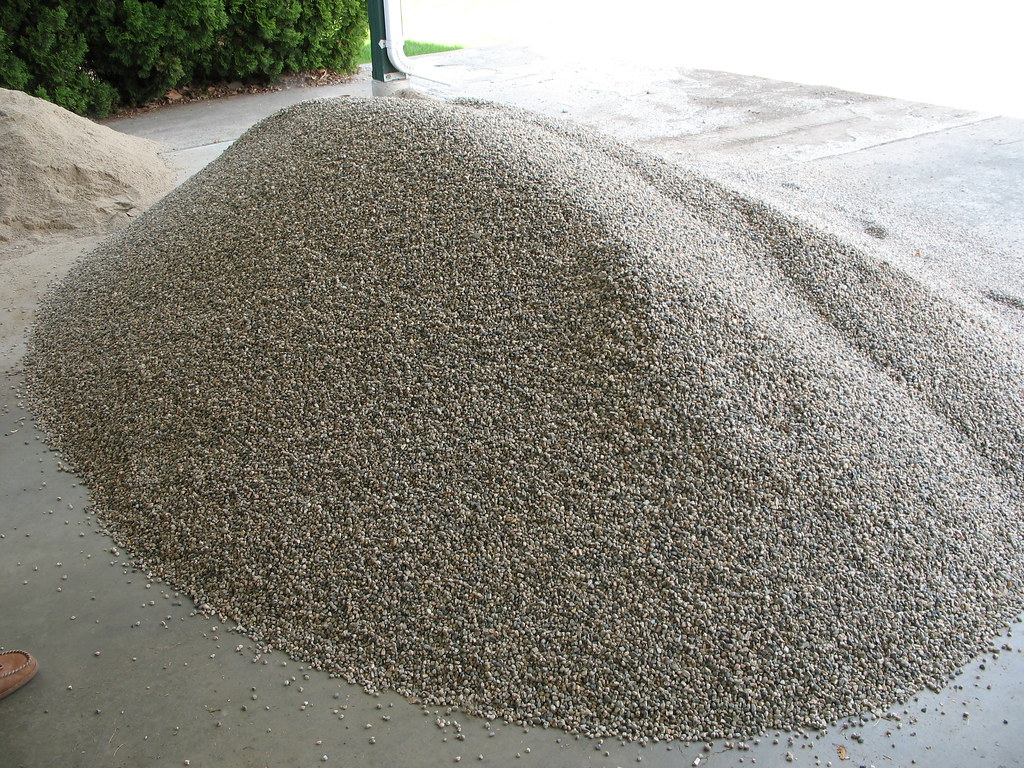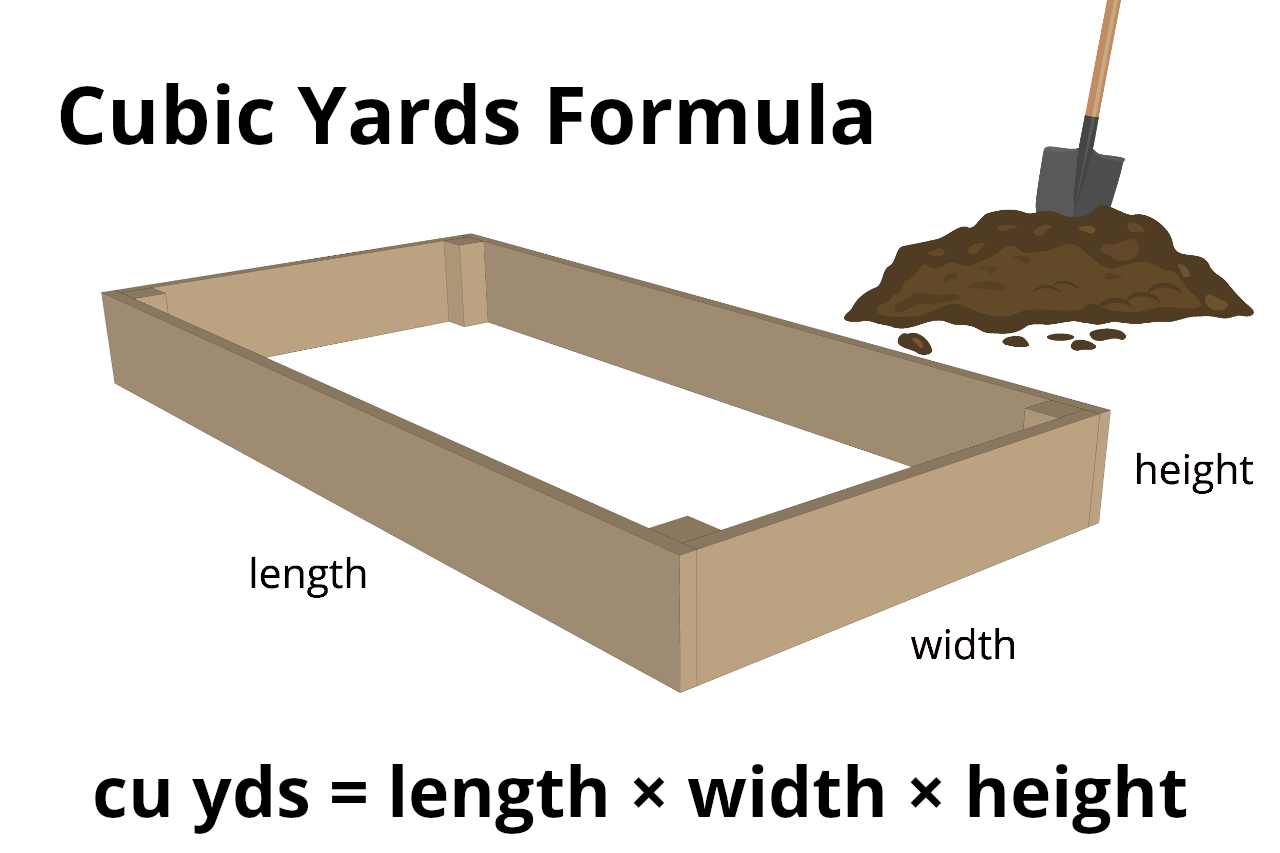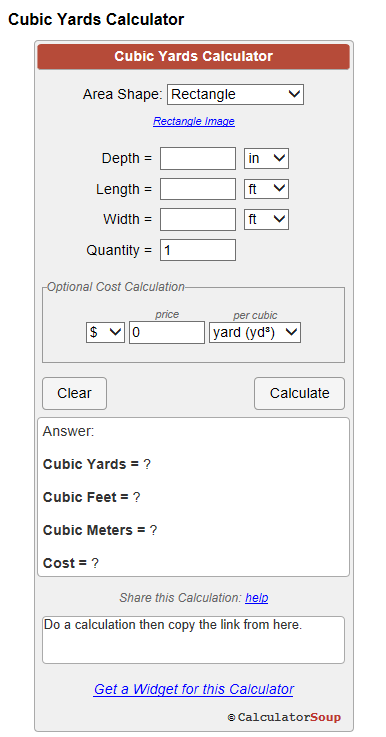# What is a cubic yard?

## Cubic yard meaning

A cubic yard (yd3) is an Imperial unit of volume used in Canada and the United States. It is defined as the volume of a cube with sides of one yard (or say 3 feet 36 inches) in length, 1 yard in width, and 1 yard in height.One cubic yard is equal to 27 cubic feet. In summary, It means that you need to divide your cubic feet by 27 to get your cubic yard. Generally speaking, the volume of two average-sized dustbins is approximately a cubic yard. Moreover, construction projects and volumes are the main areas where we can use cubic yards. Not only that, but it can also be used while buying materials.

## What is a cubic yard of concrete?

1 cubic yard of concrete is 3 feet wide by 3 feet high by 3 feet deep. (3′ x 3′ x 3′) There are 27 cubic feet in one cubic yard. Although it can vary depending upon the fact that you have a lot of sono tubes or just one large cylinder to fill. Moreover, a solid slab of concrete weighs approximately 4,050 pounds per cubic yard while a cubic yard of broken up concrete weighs around 2,025 pounds.

## What is a cubic yard of dirt?

A cubic yard of dirt is one yard or 3 feet long, wide, and high. In addition, one cubic yard contains 27 cubic feet. Now, before you think about measuring dirt using a yardstick, we have to keep something in mind. The soil will compress down if it is wet and fluff up if it is dry. Depending on how you intend to use the dirt, you can either purchase topsoil or fill dirt.## What is a cubic yard of gravel?

In the Imperial or US customary measurement system, generally, weight of 1 cubic Yard of concrete gravel is around 4050 lbs or 2.025 US tons. Moreover, the weight of 1 cubic Yard of loose and dry gravel is around 2562lbs or 1.281 US tons. In addition, a yard of gravel usually weighs about 2,200 pounds according to the UK measurement system.## What is a cubic yard of mulch?

Measuring one cubic yard of mulch is no different. In professional mulch sales, a “yard” of mulch is a volume of mulch that measures three feet by three feet by three feet, or 3′ x 3′ x 3′. We multiply the length by the width by the depth. In the same manner, that would be 3 x 3 x 3 or 27 cubic feet. Moreover, cubic yards of mulch typically weigh around 400 to 800 pounds (181 to 363 kg). Needless to say, the weight of mulch will vary upon its moisture content. Compost is usually heavier than mulch and a cubic yard of it will typically weigh between 1000 and 1600 pounds (454 to 726 kilograms).

## What is a cubic yard of stone?

A cubic yard of stone equals 3000 pounds, In addition, a short tons weighs around 2000 lbs, so number of tons,

3000/ 2000 = 1.5 short tons

In this regard, there are 1.5 short tons of stone in a cubic yard. This is the standard weight of stone in tons for one cubic yard used for billing purposes.

## What is a cubic yard of soil?

In the case of soil, measurement of 1 cubic yard depends on the amounts of the components it’s made up of. For example, one cubic yard of dry sandy soil weighs about 2,600 pounds, while on the other hand, 1 cubic yard of dry clay soil weighs in around 1,700 pounds.

## Difference between a yard and a cubic yard

A yard represents a two-dimensional area and measures only the length and width of it whereas the cubic yard covers a three-dimensional space and measures length, breadth, and height. Moreover, yards are measured in feet while the cubic yard is measured in sq feet.

## Cubic yard vs cubic meter

Cubic meter is one of the most basic measurement units for volume. It represents the volume which is equal to a cube with 1 meter at each side. Needless to say, this unit is very popular and very widely used around the world even in countries like the UK, the US, and other ones which adopted the Imperial system. One cubic meter is equal to 1,000 liters, roughly 1.31 cubic yards, or roughly 35.3 cubic feet. On the other hand, one cubic yard is equal to 27 cubic feet, roughly 764.5549 liters, and 46656 cubic inches. In order to convert one cubic meters into cubic yards we just need to multiply the cubic meter with 1.3080. In short;

 m³ =                 yd³ ______   1.3080

Cubic yard conversions

1 cubic yard is simply length, breadth and height divided by 27. A cubic yard is just one of many different units of volume or space. It is mostly used in the United States. Moreover, It is not that common, even though both Canada and the UK use it to some extent. It is because Canada and the UK have adopted the International system of Units (SI). Cubic yards may be used when discussing larger volumes, such as the volume of cement necessary to make enough concrete to build a house or the amount of fertile soil needed to completely fill a garden. In addition, dumpster volume is calculated in cubic yard due to its shape.Most of the rest of the world uses SI units of volume. The SI base unit of volume is the cubic meter, but the liter, an SI derived unit of volume, is most commonly used. In addition, there are many other measurements of volume in various systems of measurement,yet most countries use either SI, the US customary (or imperial) system, or some combination of the two. Below are the relationships between cubic yards and some commonly used units of volume in various systems of measurement.

= 27 cubic feet

= 46656 cubic inches

≈ 1.83426465×10−10 cubic miles

≈ 21.0223197 imperial bushels

≈ 21.6962157 US bushels

≈ 4.80890476 crude barrels

≈ 764.5549 litres

### What is a cubic yard to cubic meters

According to the international system, cubic yards and cubic meters are almost proportional in volume. In measurements, one cubic yard is equal to 0.76455486 m.

### What is a cubic yard to cubic foot

Yard and feet are inter-proportional to each other. If we talk about measurements, 1 cubic yard is equal to 27 cubic feet.

### What is a cubic yard to gallons

Yard and gallons are not what we usually hear as they both are quite different. Nevertheless, one cubic yard is equal to 168.178483 imperial gallons.

### What is a cubic yard to tonnes

Tonne is generally used to measure heavy weights. One cubic yard is equal to 0.27 ton reg.

### What is a cubic yard to kgs

In order to convert a quantity of a substance or material written in volume to mass we simply use the formula:

 mass = density × volume

Now convert the mass from kilograms to cubic yard;

 mass = d × v × vcf

Where vcf is the conversion factor which is used to convert cubic yards to cubic meters

So, plugging these values in the formula above, we get:

mass = 1000 × 1 × 0.764554858

or , = 1000 × 0.764554858, or

or,  = 764.555 kilograms

## How to measure cubic yard

Generally speaking, the cubic yard is a unit of volume commonly used while calculating the quantity of materials. It can be for gardening, landscaping or doing some construction work. Moreover, in order to calculate the cubic yard you need to :-### 1.    Obtain all necessary measurements in your space

Cubic yard volumes can be obtained relatively easily for a variety of three-dimensional spaces via a few simple calculations. Even though it is easy to take these measurements in feet or inches, these equations need that all measurements have been taken in yards. Thus, before using one of these equations, it’s important to ensure that you took your initial measurement(s) in yards or, alternatively, that you converted them into yards via a conversion factor. In short, make sure your end result is in the yard and not in any other unit. A few conversions for common measurements of length are below:

1 yard = 3 feet

= 36 inches

= 0.914 meters

= 91.44 centimeters

### 2.    Measure the volume required space

Once you are done with the basic measurements in yards, it is time to calculate the volumetric measurement as it is the easiest way to measure the cubic yard. Also if your calculations are in any other kind of unit; let’s suppose it is in feet. In this situation, you first measure the volume in feet and then divide it by 27. For example, suppose you need to measure your bedroom in a cubic yard. The measurements of your room are 12 ft in length, 12 ft in width, and 9 ft in height. Now, in order to calculate the volume we just multiply the

Volume = 12 x 12 x 9

= 144 x 9

= 1296 sq feet.

Now, in order to calculate the cubic yard we divide the calculated volume by 27:-

= 1296/27

= 48 cubic yard or  yd3

Hence, this is how you calculate the cubic yard. Moreover, there are multiple ways you can measure that in the case of different shapes such as-

### Use the equation L × W × H for rectangular spaces

The volume of any rectangular three-dimensional space such as rectangular prism,cube, or cuboid can be easily measured by multiplying its length times its width times its height. In short, multiply all three of its dimensions. In other words, this equation can also be called as multiplying the surface area of one of the rectangular space’s faces by the dimension perpendicular to that face of it. Read Also: Cups to grams: a measurement for baking

For example, let’s say we want to determine the volume (in yd3) of the study room in our house. We measure the study room to be 5 yd long, 4 yd wide, and 3 yd high.now in order to calculate the volumetric measurement, we just need to multiply its length, width, and height like for example;

5 × 4 × 3

= 20 × 3

= 60.

Henceforth we can say that the study room has a volume of 30 cubic yard or 30 yd3.

Since cubes are rectangular spaces where all sides have equal measurements. Therefore, you can change the equation to measure the volume of a from L × W × H to a3

### Use the equation π × R2 × h for cylindrical spaces

In order to find the volume of a cylindrical space we simply need to multiply the two-dimensional area of one of its circular faces by the height of the cylinder. Firstly, we need to find the area of its circular face by using the circular area equation, in other words, multiply the mathematical constant π  or pi (whose mathematical value stands around 3.1459) by the radius of the circle, or say, the distance from the center of the circle to one of its edges. At last, all we need to do is simply multiply the measured area with the provided height of the cylinder. Given these points, always ensure that all values are in yards and not in some different unit.

For example, let’s suppose we want to determine the volume of a cylindrical hole in our back yard in order to make a swimming pool. The hole is 2 yards in diameter and 3 yards in depth. Now we need to divide the distance of the hole in half to get the pool’s radius which will be 1 yard. Then, simply put the measurements in the cylindrical equation and you will get:

(3.14159) × 12 × 3

= 1 x (3.14159) × 3

= 9.42477. Therefore the hole has a volume of 9.42477 cubic yards or in simple words,9.42 yd3.

### Use equation 4/3 π x  R3  in the case of spheres

For spheres,you need to use the equation 4/3 pi × R3 . In order to measure the volume of a sphere in cubic yards, firstly you need to know its radius, or say the distance from its center to its outside edge, preferably in yards. Coupled to that you have to cube this number (multiply it by itself 3 times), and then multiply it by 4/3 x 3.14159 to get the volume of the sphere in cubic yards.

Let’s clear this up through an example. Now suppose we want to find the volume inside a huge spherical rubber ball . The ball is 5 yards across. Divide 5 yards in half to find the balloon’s radius which will be 2.5 yards. Now put this calculated value into the equation as follows:

= 4/3 pi × (2.5)3

= 4/3 (3.14159) × 15.625

= 4.189 × 15.625

= 65.4

Therefore, the volume of the ball is 65.4 cubic yard or 65.4 yd3.

### ⅓ pi x R2 × H for cones

In the case of cones, we need to use the equation 1/3 pi × R2 × H as mentioned above.. We just need to find the height and radius of the preferred cone (in yards), and then calculate the cubic yard simply as we calculated the volume of the cylinder. But make sure that we need to multiply our result by 1/3 to get the volume of the cone.

Again, let’s learn it properly with an example. Suppose that we want to find the volume of a birthday hat, one we usually wear on birthday parties. It has a radius of approximately 20 cm or say, 0.21 yards. Moreover it has a height of around 30 cm or 0.32 yards. Putting these measured numbers into the equation and solving it gives us:

= 1/3 (3.14159) × 0.21² × .32

= 1/3 (3.14159) × 0.0441 × .32

= 1.04 x 0.014112

= 146.7 x 10-4.

Therefore there is 146.7 x 10-4 yd3 in the birthday cap.

## What is a cubic yard and where is it used

Construction areas are the major areas where cubic yards are used. Most common things we can measure using cubic yard are concrete

Cement, sand, aggregate, rock, asphalt, gravel, dirt, compost, mulch and topsoil.## What is a cubic yard calculator

There are times when you need to calculate the cubic yard as soon as you can. In these kinds of situations, a cubic yard calculator comes in handy. Cubic yards calculators come in handy during construction work, especially when you need to measure the volume for landscape material, mulch, fill, gravel, cement or containers. Needless to say, cubic yards calculators are massively used in construction sites.

## How to use the calculatorFirstly, enter dimensions in US units (inches or feet) or metric units (centimeters or meters) to calculate the cubic yards, cubic feet and cubic meters.

Enter measurements in US units or metric units. Calculate volume from your measurements in inches (in), feet (ft), yards (yd), centimeters (cm), or meters (m). In addition, you can also enter decimal values. For example, if you have one measurement that is 7’3″ you can enter that as 7.25 feet instead. Moreover, If you have a measurement of 245 cm you can also enter that as 2.45 m.

Even though you have an area in square feet you can still calculate cubic yards. In the calculator, select Rectangle. In addition to that, enter your square feet in the Length field, and enter “1” in the Width field. Enter how deep you want the fill in the Depth field.

## FAQs related to cubic yard

### 1.   How do you calculate cubic yards?

Length in feet x width in feet x height or depth in feet divided by 27 is equal to one cubic yard. Moreover, In the case of inches we need to further divide it by 12.

### 2.   What is a cubic yard?

A cubic yard is the space which contains the length, width and depth of one yard (more preferably 3 feet or 36 inches).

### 3.   What exactly does cubic yard mean?

Cubic yard is a unit of volume which carries one yard in each of the dimensions of a three-dimensional figure.

### 4.   How many yards of concrete do I need for a 24 x 24 slab?

Suppose that the space is 4 yards deep. Now, in order to fill that we need a concrete slab that is 24 x 24 x 4. In addition to that, simply put these measurements in the cubic calculator and we will get our answer. The answer here is 7.11 yards.

### 5.   How do I calculate how much cubic yards of stone I need?

It is fairly simple; just multiply the length, width and height of the preferred space and divide it by 27. This will exactly tell us the amount of stone or the amount of crushed stone needed.

### 6.   Is one yard and one cubic yard the same?

According to the US system, 1 yard is equal to 3 feet or 3 inches. A cubic yard is a space that acquires length, width, and depth of one yard each.